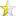### C++

Dec 7, 2013 (last update: Dec 8, 2013)

Score: 3.6/5 (1098 votes)A set of items of the same type in which each item points to ("is linked to") the next item in the list. Ordering of items is not part of the definition, therefore, we will not consider the ordering. Yet determined according to the usage.

NOTE: Since sequence of elements is not part of definition of a linked list, many other structures can be implemented using Linked Lists.
E.g. if items are ordered according to the sequence of insertion into the list, this corresponds to a stack, in which the top item is pointed by the list head pointer.

• List head is a special pointer to the first item in the list.
• The last node(rear) points to a NULL address
• In processing a list, any node can only be accessed after having accessed all other nodes before it. This property might also be called, in other words, Strict Sequential Access (SSA).

•  ``123456789101112131415161718192021222324252627282930313233343536373839404142434445464748495051`` ``````// implementation of LinkedList // the Node class will be given later // Author: Ali Selcuk AKYUZ // Mail: selcuk@retinarobotics.com || e174043@metu.edu.tr // Electrical and Electronics Engineering Department // Middle East Technical University - ANKARA // If any questions please send me an email #include #include "Node.cpp" using namespace std; int main () { Node *p,*q,*r; // Link the nodes with each other q = new Node('B'); // here nxtptr is passed by a nullptr by default p = new Node('A',q); r = new Node('C'); // modify the list q->InsertAfter(r); /* Call the InsertAfter method that belongs to the object pointed by q, as paramater, pass to it the address contained in r. */ cout << "p:" << p->data << endl; // "A" will be printed out cout << "p_next:" << p->NextNode()->data << endl; // "B" will be printed out cout << "q:" << q->data << endl; // "B" will be printed out cout << "q_next:" << q->NextNode()->data << endl; // "C" will be printed out cout << "r:" << r->data << endl; // "C" will be printed out p = p->NextNode(); // p now points to the node coming after the node it was // previously pointing to. cout << endl; cout << "p:" << p->data << endl; // "B" will be printed out r = q->DeleteAfter(); // copy to r the address of the node pointed by //the node pointed by the node pointed by q, and remove that node from the list Node *head; head = GetNode('A',GetNode('B',GetNode('C'))); /* Here above method, creates a list which has nodes having data A,B,C and each node pointing to the next one respectively. */ delete q; delete p; delete r; return 0; }``````

When we compile and run this program, the screen will show:
 ``` p:A P_next:B q:B q_next:C r:C p:B ```

Now let us implement the Node class so that we can have a better understanding of this structure.

 ``1234567891011121314151617181920212223242526272829303132`` ``````// Node.h // Author: Ali Selcuk AKYUZ // Mail: selcuk@retinarobotics.com || e174043@metu.edu.tr // Electrical and Electronics Engineering Department // Middle East Technical University - ANKARA // If any questions please send me an email #ifndef NODE_H #define NODE_H #include using namespace std; template class Node { public: Node(); Node(const T& item, Node* ptrnext = NULL); T data; // access to the next node Node* NextNode(); // list modification methods void InsertAfter(Node* p); Node* DeleteAfter(); Node * GetNode(const T& item, Node* nextptr = NULL); private: Node * next; }; #endif // NODE_H ``````

Here we have a default constructer, and three methods that will be explained later in the cpp part of the class implementation.

 ``12345678910111213141516171819202122232425262728293031323334353637383940414243444546474849505152535455565758596061626364656667686970`` ``````// implementation of Node class // Author: Ali Selcuk AKYUZ // Mail: selcuk@retinarobotics.com || e174043@metu.edu.tr // Electrical and Electronics Engineering Department // Middle East Technical University - ANKARA // If any questions please send me an email #include "Node.h" template Node::Node() { // default constructor // this is to allow us to create an object without any initialization } // This constructor is just to set next pointer of a node and the data contained. template Node::Node(const T& item,Node* ptrnext) { this->data = item; this->next = ptrnext; } template Node*Node::NextNode() { return this->next; } // This methods inserts a node just after the node that the method belongs to // TO-DO: Consider a better implementation template void Node::InsertAfter(Node *p) { // not to lose the rest of the list, we ought to link the rest of the // list to the Node* p first p->next = this->next; // now we should link the previous Node to Node *p , i.e the Node that we are //inserting after, this->next = p; } // Deletes the node from the list and returns the deleted node template Node* Node::DeleteAfter() { // store the next Node in a temporary Node Node* tempNode = next; // check if there is a next node if(next != NULL) next = next->next; return tempNode; } template Node * GetNode(const T& item, Node* nextptr = NULL) { Node* newnode; // Local ptr for new node newnode = new Node(item,nextptr); if ( newnode == NULL) { cerr << "Memory allocation failed." << endl; exit(1); } return newnode; }``````

After implementing the node class, now we can implement stacks, queues and the like. Let me implement these structures by using Linked List logic.

### Stack

If the items are ordered according to the sequence of insertion into the list, this corresponds to a stack.In other words, First In Last Out (FILO) or Last In First Out (LIFO)

### Queue

A queue is a data structure consisting of a list of items and two pointers to the "front" and "rear" items in the list. Items can only inserted at the rear and removed only at the front. i.e. FIFO (First In First Out) operation.

I will implement these classes in another article.

ENJOY!!!!